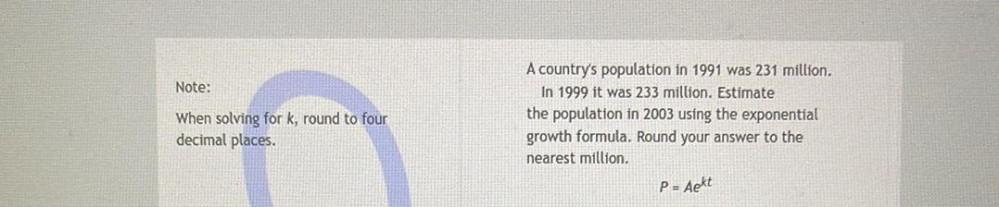Question:

# Note: When solving for k, round to four decimal places. A country's population in 1991 was 231 million. In 1999 it was 233 milliNote: When solving for k, round to four decimal places. A country's population in 1991 was 231 million. In 1999 it was 233 million. Estimate the population in 2003 using the exponential growth formula. Round your answer to the nearest million, P= Aekt